PSAT Math : Polynomials

Example Questions

1 2 3 5 Next →

Example Question #12 : Trinomials

Find the sum: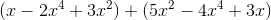Write the answer in standard form.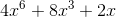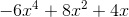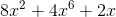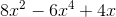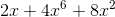Explanation:

Find the sum:Write the answer in standard form.

Combine like terms: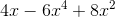Write the answer in standard form (terms with the highest degree first):Example Question #74 : Expressions

Evaluate the following: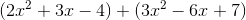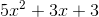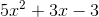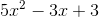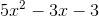Explanation:

With this problem, you need to take the trinomials out of parentheses and combine like terms. Since the two trinomials are being added together, you can remove the parentheses without needing to change any signs: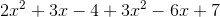The next step is to combine like terms, based on the variables. You have two terms with, two terms with, and two terms with no variable. Make sure to pay attention to plus and minus signs with each term when combining like terms:Example Question #75 : Expressions

Evaluate the following: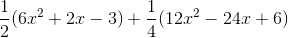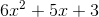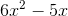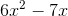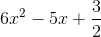Explanation:

With this problem, you need to distribute the two fractions across each of the trinomials. To do this, you multiply each term inside the parentheses by the fraction outside of it: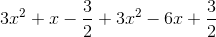The next step is to combine like terms, based on the variables. You have two terms with, two terms with, and two terms with no variable. Make sure to pay attention to plus and minus signs with each term when combining like terms. Since you have a positive and negative, those two terms will cancel out:Example Question #13 : Trinomials

Evaluate the following: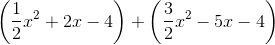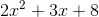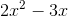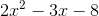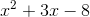Explanation:

To add these two trinomials, you will first begin by combining like terms. You have two terms with, two terms with, and two terms with no variable. For the two fractions with, you can immediately add because they have common denominators: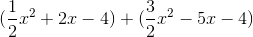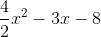Example Question #14 : Trinomials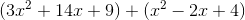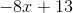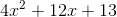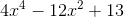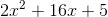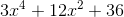Explanation:

To add trinomials, identify and group together the like-terms: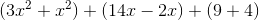. Next, factor out what is common between the like-terms: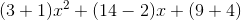. Finally, add what is left inside the parentheses to obtain the final answer of.

Example Question #6 : Simplifying Polynomials

Evaluate the following: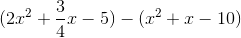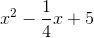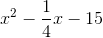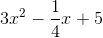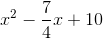Explanation:

To subtract these two trinomials, you first need to flip the sign on every term in the second trinomial, since it is being subtrated: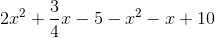Next you can combine like terms. You have two terms with, two terms with, and two terms with no variable:Example Question #11 : Trinomials

Find the difference: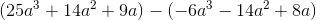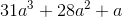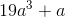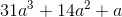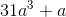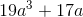Explanation:

Find the difference:Distribute the negative to the second trinomial: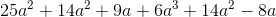Combine like terms:Example Question #1 : How To Subtract Trinomials

Subtract: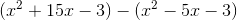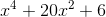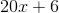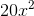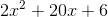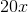Explanation:

When subtracting trinomials, first distribute the negative sign to the expression being subtracted, and then remove the parentheses: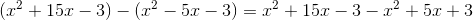Next, identify and group the like terms in order to combine them: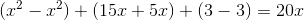.

Example Question #1 : How To Divide Trinomials

Find the quotient: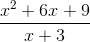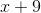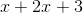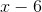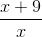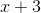Explanation:

Find the quotient:Step one: Factor the numerator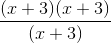Step two: Simplify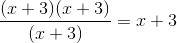1 2 3 5 Next →